# 8.3 Inverse trigonometric functions  (Page 9/15)

 Page 9 / 15

What are the amplitude, period, and phase shift for the function?

amplitude: 8,000; period: 10; phase shift: 0

Over this domain, when does the population reach 18,000? 13,000?

What is the predicted population in 2007? 2010?

In 2007, the predicted population is 4,413. In 2010, the population will be 11,924.

For the following exercises, suppose a weight is attached to a spring and bobs up and down, exhibiting symmetry.

Suppose the graph of the displacement function is shown in [link] , where the values on the x -axis represent the time in seconds and the y -axis represents the displacement in inches. Give the equation that models the vertical displacement of the weight on the spring.

At time = 0, what is the displacement of the weight?

5 in.

At what time does the displacement from the equilibrium point equal zero?

What is the time required for the weight to return to its initial height of 5 inches? In other words, what is the period for the displacement function?

10 seconds

## Inverse Trigonometric Functions

For the following exercises, find the exact value without the aid of a calculator.

${\mathrm{sin}}^{-1}\left(1\right)$

${\mathrm{cos}}^{-1}\left(\frac{\sqrt{3}}{2}\right)$

$\frac{\pi }{6}$

${\mathrm{tan}}^{-1}\left(-1\right)$

${\mathrm{cos}}^{-1}\left(\frac{1}{\sqrt{2}}\right)$

$\frac{\pi }{4}$

${\mathrm{sin}}^{-1}\left(\frac{-\sqrt{3}}{2}\right)$

${\mathrm{sin}}^{-1}\left(\mathrm{cos}\left(\frac{\pi }{6}\right)\right)$

$\frac{\pi }{3}$

${\mathrm{cos}}^{-1}\left(\mathrm{tan}\left(\frac{3\pi }{4}\right)\right)$

$\mathrm{sin}\left({\mathrm{sec}}^{-1}\left(\frac{3}{5}\right)\right)$

No solution

$\mathrm{cot}\left({\mathrm{sin}}^{-1}\left(\frac{3}{5}\right)\right)$

$\mathrm{tan}\left({\mathrm{cos}}^{-1}\left(\frac{5}{13}\right)\right)$

$\frac{12}{5}$

$\mathrm{sin}\left({\mathrm{cos}}^{-1}\left(\frac{x}{x+1}\right)\right)$

Graph $\text{\hspace{0.17em}}f\left(x\right)=\mathrm{cos}\text{\hspace{0.17em}}x\text{\hspace{0.17em}}$ and $\text{\hspace{0.17em}}f\left(x\right)=\mathrm{sec}\text{\hspace{0.17em}}x\text{\hspace{0.17em}}$ on the interval $\text{\hspace{0.17em}}\left[0,2\pi \right)\text{\hspace{0.17em}}$ and explain any observations.

The graphs are not symmetrical with respect to the line $\text{\hspace{0.17em}}y=x.\text{\hspace{0.17em}}$ They are symmetrical with respect to the $\text{\hspace{0.17em}}y$ -axis.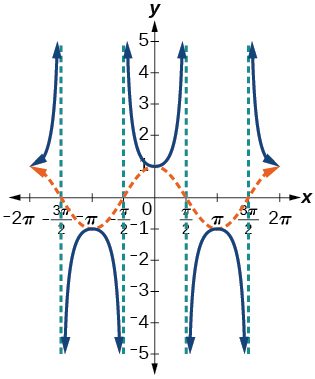Graph $\text{\hspace{0.17em}}f\left(x\right)=\mathrm{sin}\text{\hspace{0.17em}}x\text{\hspace{0.17em}}$ and $\text{\hspace{0.17em}}f\left(x\right)=\mathrm{csc}\text{\hspace{0.17em}}x\text{\hspace{0.17em}}$ and explain any observations.

Graph the function $f\text{\hspace{0.17em}}\left(x\right)=\frac{x}{1}-\frac{{x}^{3}}{3!}+\frac{{x}^{5}}{5!}-\frac{{x}^{7}}{7!}\text{\hspace{0.17em}}$ on the interval $\text{\hspace{0.17em}}\left[-1,1\right]\text{\hspace{0.17em}}$ and compare the graph to the graph of $\text{\hspace{0.17em}}f\left(x\right)=\mathrm{sin}\text{\hspace{0.17em}}x\text{\hspace{0.17em}}$ on the same interval. Describe any observations.

The graphs appear to be identical.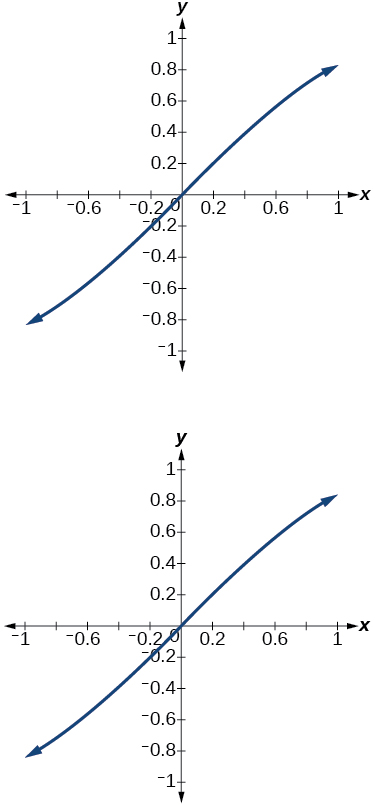## Chapter practice test

For the following exercises, sketch the graph of each function for two full periods. Determine the amplitude, the period, and the equation for the midline.

$f\left(x\right)=0.5\mathrm{sin}\text{\hspace{0.17em}}x$

amplitude: 0.5; period: $\text{\hspace{0.17em}}2\pi ;\text{\hspace{0.17em}}$ midline $\text{\hspace{0.17em}}y=0\text{\hspace{0.17em}}$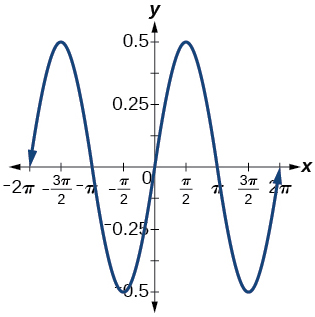$f\left(x\right)=5\mathrm{cos}\text{\hspace{0.17em}}x$

$f\left(x\right)=5\mathrm{sin}\text{\hspace{0.17em}}x$

amplitude: 5; period: $\text{\hspace{0.17em}}2\pi ;\text{\hspace{0.17em}}$ midline: $\text{\hspace{0.17em}}y=0$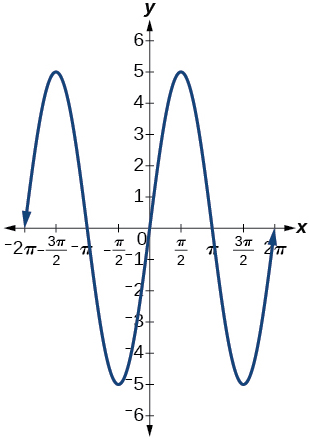$f\left(x\right)=\mathrm{sin}\left(3x\right)$

$f\left(x\right)=-\mathrm{cos}\left(x+\frac{\pi }{3}\right)+1$

amplitude: 1; period: $\text{\hspace{0.17em}}2\pi ;\text{\hspace{0.17em}}$ midline: $\text{\hspace{0.17em}}y=1$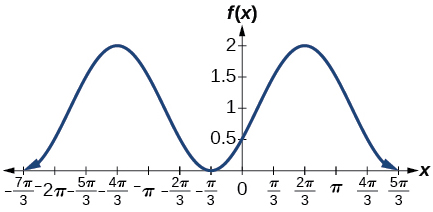$f\left(x\right)=5\mathrm{sin}\left(3\left(x-\frac{\pi }{6}\right)\right)+4$

$f\left(x\right)=3\mathrm{cos}\left(\frac{1}{3}x-\frac{5\pi }{6}\right)$

amplitude: 3; period: $\text{\hspace{0.17em}}6\pi ;\text{\hspace{0.17em}}$ midline: $\text{\hspace{0.17em}}y=0$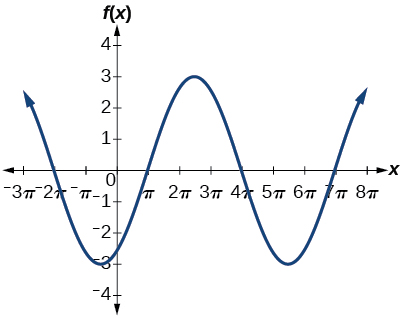$f\left(x\right)=\mathrm{tan}\left(4x\right)$

$f\left(x\right)=-2\mathrm{tan}\left(x-\frac{7\pi }{6}\right)+2$

amplitude: none; period: midline: asymptotes: where is an integer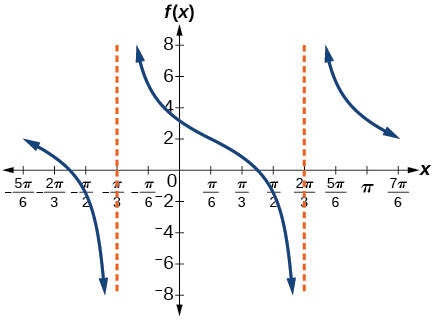$f\left(x\right)=\pi \mathrm{cos}\left(3x+\pi \right)$

$f\left(x\right)=5\mathrm{csc}\left(3x\right)$

amplitude: none; period: midline: asymptotes: where is an integer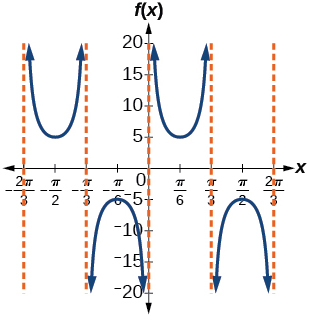$f\left(x\right)=\pi \mathrm{sec}\left(\frac{\pi }{2}x\right)$

$f\left(x\right)=2\mathrm{csc}\left(x+\frac{\pi }{4}\right)-3$

amplitude: none; period: $\text{\hspace{0.17em}}2\pi ;\text{\hspace{0.17em}}$ midline: $\text{\hspace{0.17em}}y=-3$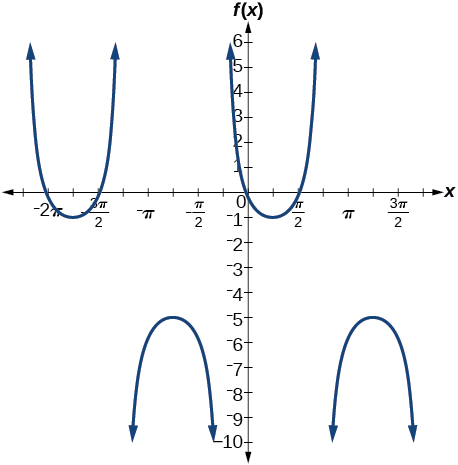For the following exercises, determine the amplitude, period, and midline of the graph, and then find a formula for the function.

Give in terms of a sine function.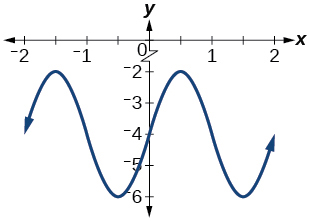Give in terms of a sine function.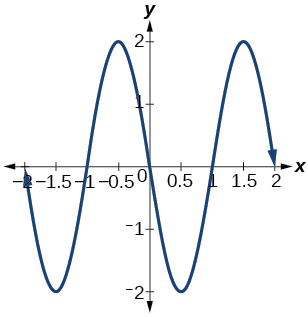amplitude: 2; period: 2; midline: $\text{\hspace{0.17em}}y=0;$ $f\left(x\right)=2\mathrm{sin}\left(\pi \left(x-1\right)\right)$

Give in terms of a tangent function.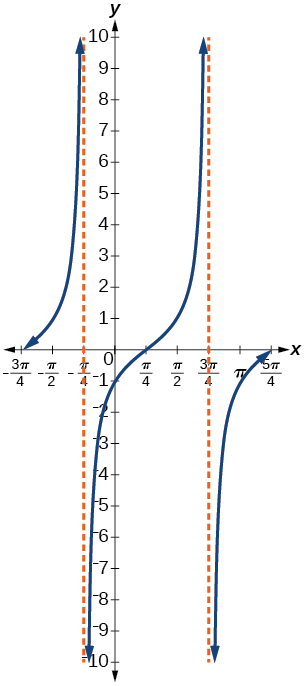For the following exercises, find the amplitude, period, phase shift, and midline.

$y=\mathrm{sin}\left(\frac{\pi }{6}x+\pi \right)-3$

amplitude: 1; period: 12; phase shift: $\text{\hspace{0.17em}}-6;\text{\hspace{0.17em}}$ midline $\text{\hspace{0.17em}}y=-3$

$y=8\mathrm{sin}\left(\frac{7\pi }{6}x+\frac{7\pi }{2}\right)+6$

The outside temperature over the course of a day can be modeled as a sinusoidal function. Suppose you know the temperature is 68°F at midnight and the high and low temperatures during the day are 80°F and 56°F, respectively. Assuming $\text{\hspace{0.17em}}t\text{\hspace{0.17em}}$ is the number of hours since midnight, find a function for the temperature, $\text{\hspace{0.17em}}D,\text{\hspace{0.17em}}$ in terms of $\text{\hspace{0.17em}}t.$

$D\left(t\right)=68-12\mathrm{sin}\left(\frac{\pi }{12}x\right)$

Water is pumped into a storage bin and empties according to a periodic rate. The depth of the water is 3 feet at its lowest at 2:00 a.m. and 71 feet at its highest, which occurs every 5 hours. Write a cosine function that models the depth of the water as a function of time, and then graph the function for one period.

For the following exercises, find the period and horizontal shift of each function.

$g\left(x\right)=3\mathrm{tan}\left(6x+42\right)$

period: $\text{\hspace{0.17em}}\frac{\pi }{6};\text{\hspace{0.17em}}$ horizontal shift: $\text{\hspace{0.17em}}-7$

$n\left(x\right)=4\mathrm{csc}\left(\frac{5\pi }{3}x-\frac{20\pi }{3}\right)$

Write the equation for the graph in [link] in terms of the secant function and give the period and phase shift.

$f\left(x\right)=\mathrm{sec}\left(\pi x\right);\text{\hspace{0.17em}}$ period: 2; phase shift: 0

If $\text{\hspace{0.17em}}\mathrm{tan}\text{\hspace{0.17em}}x=3,\text{\hspace{0.17em}}$ find $\text{\hspace{0.17em}}\mathrm{tan}\left(-x\right).$

If $\text{\hspace{0.17em}}\mathrm{sec}\text{\hspace{0.17em}}x=4,\text{\hspace{0.17em}}$ find $\text{\hspace{0.17em}}\mathrm{sec}\left(-x\right).$

$4$

For the following exercises, graph the functions on the specified window and answer the questions.

Graph $\text{\hspace{0.17em}}m\left(x\right)=\mathrm{sin}\left(2x\right)+\mathrm{cos}\left(3x\right)\text{\hspace{0.17em}}$ on the viewing window $\text{\hspace{0.17em}}\left[-10,10\right]\text{\hspace{0.17em}}$ by $\text{\hspace{0.17em}}\left[-3,3\right].\text{\hspace{0.17em}}$ Approximate the graph’s period.

Graph $\text{\hspace{0.17em}}n\left(x\right)=0.02\mathrm{sin}\left(50\pi x\right)\text{\hspace{0.17em}}$ on the following domains in $\text{\hspace{0.17em}}x:$ $\left[0,1\right]\text{\hspace{0.17em}}$ and $\text{\hspace{0.17em}}\left[0,3\right].\text{\hspace{0.17em}}$ Suppose this function models sound waves. Why would these views look so different?

The views are different because the period of the wave is $\text{\hspace{0.17em}}\frac{1}{25}.\text{\hspace{0.17em}}$ Over a bigger domain, there will be more cycles of the graph.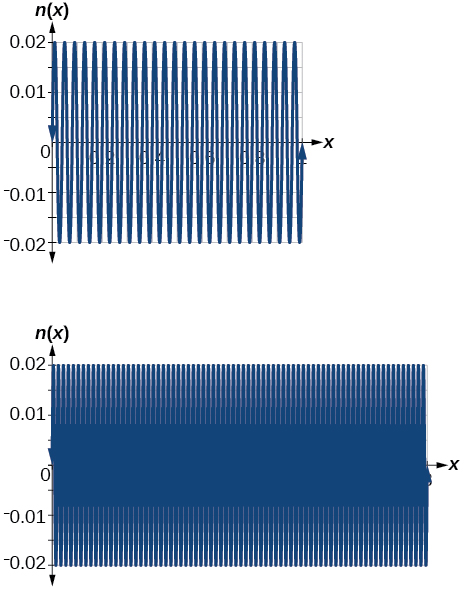Graph $\text{\hspace{0.17em}}f\left(x\right)=\frac{\mathrm{sin}\text{\hspace{0.17em}}x}{x}\text{\hspace{0.17em}}$ on $\text{\hspace{0.17em}}\left[-0.5,0.5\right]\text{\hspace{0.17em}}$ and explain any observations.

For the following exercises, let $\text{\hspace{0.17em}}f\left(x\right)=\frac{3}{5}\mathrm{cos}\left(6x\right).$

What is the largest possible value for $\text{\hspace{0.17em}}f\left(x\right)?$

$\frac{3}{5}$

What is the smallest possible value for $\text{\hspace{0.17em}}f\left(x\right)?$

Where is the function increasing on the interval $\text{\hspace{0.17em}}\left[0,2\pi \right]?$

On the approximate intervals $\text{\hspace{0.17em}}\left(0.5,1\right),\left(1.6,2.1\right),\left(2.6,3.1\right),\left(3.7,4.2\right),\left(4.7,5.2\right),\left(5.6,6.28\right)$

For the following exercises, find and graph one period of the periodic function with the given amplitude, period, and phase shift.

Sine curve with amplitude 3, period $\text{\hspace{0.17em}}\frac{\pi }{3},\text{\hspace{0.17em}}$ and phase shift $\text{\hspace{0.17em}}\left(h,k\right)=\left(\frac{\pi }{4},2\right)$

Cosine curve with amplitude 2, period $\text{\hspace{0.17em}}\frac{\pi }{6},\text{\hspace{0.17em}}$ and phase shift $\text{\hspace{0.17em}}\left(h,k\right)=\left(-\frac{\pi }{4},3\right)$

$f\left(x\right)=2\mathrm{cos}\left(12\left(x+\frac{\pi }{4}\right)\right)+3$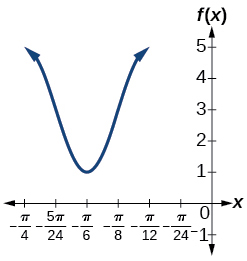For the following exercises, graph the function. Describe the graph and, wherever applicable, any periodic behavior, amplitude, asymptotes, or undefined points.

$f\left(x\right)=5\mathrm{cos}\left(3x\right)+4\mathrm{sin}\left(2x\right)$

$f\left(x\right)={e}^{\mathrm{sin}t}$

This graph is periodic with a period of $\text{\hspace{0.17em}}2\pi .$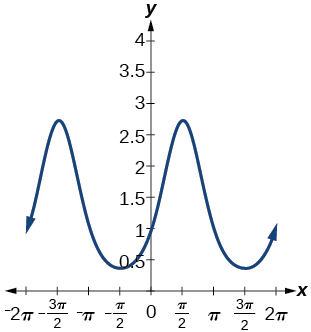For the following exercises, find the exact value.

${\mathrm{sin}}^{-1}\left(\frac{\sqrt{3}}{2}\right)$

${\mathrm{tan}}^{-1}\left(\sqrt{3}\right)$

$\frac{\pi }{3}$

${\mathrm{cos}}^{-1}\left(-\frac{\sqrt{3}}{2}\right)$

${\mathrm{cos}}^{-1}\left(\mathrm{sin}\left(\pi \right)\right)$

$\frac{\pi }{2}$

${\mathrm{cos}}^{-1}\left(\mathrm{tan}\left(\frac{7\pi }{4}\right)\right)$

$\mathrm{cos}\left({\mathrm{sin}}^{-1}\left(1-2x\right)\right)$

$\sqrt{1-{\left(1-2x\right)}^{2}}$

${\mathrm{cos}}^{-1}\left(-0.4\right)$

$\mathrm{cos}\left({\mathrm{tan}}^{-1}\left({x}^{2}\right)\right)$

$\frac{1}{\sqrt{1+{x}^{4}}}$

For the following exercises, suppose $\text{\hspace{0.17em}}\mathrm{sin}\text{\hspace{0.17em}}t=\frac{x}{x+1}.$

$\mathrm{tan}\text{\hspace{0.17em}}t$

$\mathrm{csc}\text{\hspace{0.17em}}t$

$\frac{x+1}{x}$

Given [link] , find the measure of angle $\text{\hspace{0.17em}}\theta \text{\hspace{0.17em}}$ to three decimal places. Answer in radians.

For the following exercises, determine whether the equation is true or false.

$\mathrm{arcsin}\left(\mathrm{sin}\left(\frac{5\pi }{6}\right)\right)=\frac{5\pi }{6}$

False

$\mathrm{arccos}\left(\mathrm{cos}\left(\frac{5\pi }{6}\right)\right)=\frac{5\pi }{6}$

The grade of a road is 7%. This means that for every horizontal distance of 100 feet on the road, the vertical rise is 7 feet. Find the angle the road makes with the horizontal in radians.

root under 3-root under 2 by 5 y square
The sum of the first n terms of a certain series is 2^n-1, Show that , this series is Geometric and Find the formula of the n^th
cosA\1+sinA=secA-tanA
why two x + seven is equal to nineteen.
The numbers cannot be combined with the x
Othman
2x + 7 =19
humberto
2x +7=19. 2x=19 - 7 2x=12 x=6
Yvonne
because x is 6
SAIDI
what is the best practice that will address the issue on this topic? anyone who can help me. i'm working on my action research.
simplify each radical by removing as many factors as possible (a) √75
how is infinity bidder from undefined?
what is the value of x in 4x-2+3
give the complete question
Shanky
4x=3-2 4x=1 x=1+4 x=5 5x
Olaiya
hi can you give another equation I'd like to solve it
Daniel
what is the value of x in 4x-2+3
Olaiya
if 4x-2+3 = 0 then 4x = 2-3 4x = -1 x = -(1÷4) is the answer.
Jacob
4x-2+3 4x=-3+2 4×=-1 4×/4=-1/4
LUTHO
then x=-1/4
LUTHO
4x-2+3 4x=-3+2 4x=-1 4x÷4=-1÷4 x=-1÷4
LUTHO
A research student is working with a culture of bacteria that doubles in size every twenty minutes. The initial population count was  1350  bacteria. Rounding to five significant digits, write an exponential equation representing this situation. To the nearest whole number, what is the population size after  3  hours?
v=lbh calculate the volume if i.l=5cm, b=2cm ,h=3cm
Need help with math
Peya
can you help me on this topic of Geometry if l help you
litshani
( cosec Q _ cot Q ) whole spuare = 1_cosQ / 1+cosQ
A guy wire for a suspension bridge runs from the ground diagonally to the top of the closest pylon to make a triangle. We can use the Pythagorean Theorem to find the length of guy wire needed. The square of the distance between the wire on the ground and the pylon on the ground is 90,000 feet. The square of the height of the pylon is 160,000 feet. So, the length of the guy wire can be found by evaluating √(90000+160000). What is the length of the guy wire?
the indicated sum of a sequence is known as
how do I attempted a trig number as a starter
cos 18 ____ sin 72 evaluateByBy Rohini AjayBy OpenStaxBy Rohini AjayBy Charles JumperBy George TurnerBy Jemekia WeedenBy Brenna FikeBy OpenStaxBy Rohini AjayBy Madison Christian